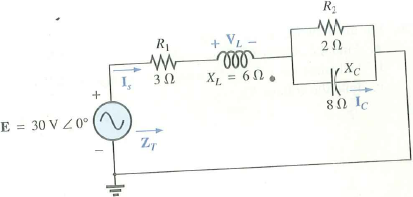Problem

# For Problem, use a frequency of 1 kHz to determine the inductive and capacitive levels req...

For Problem, use a frequency of 1 kHz to determine the inductive and capacitive levels required for the input files. In this case, write the required input file.

Do Problem 1 using PSpice or Multisim.

Problem 1

For the network in Fig. 1:

a. Find the total impedance ZT.

b. Determine the current Is.

c. Calculate IC using the current divider rule.

d. Calculate VL using the voltage divider rule.

FIG. 1#### Step-by-Step Solution

Request Professional Solution

#### Request Solution!

We need at least 10 more requests to produce the solution.

0 / 10 have requested this problem solution

The more requests, the faster the answer.

All students who have requested the solution will be notified once they are available.